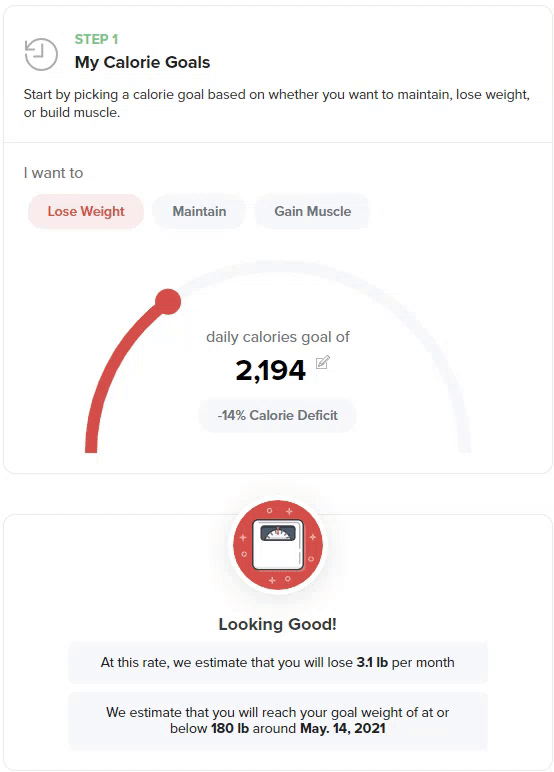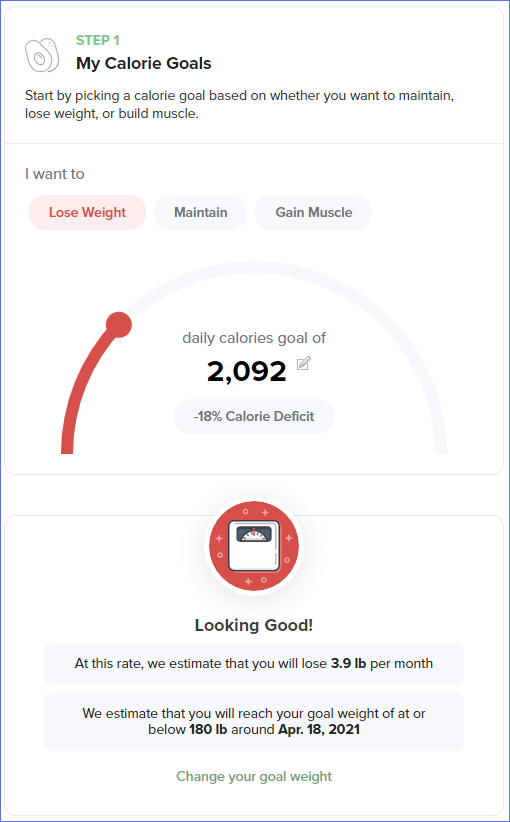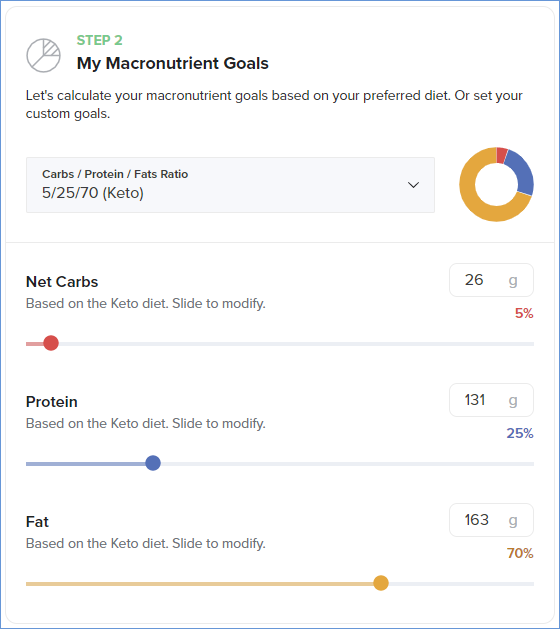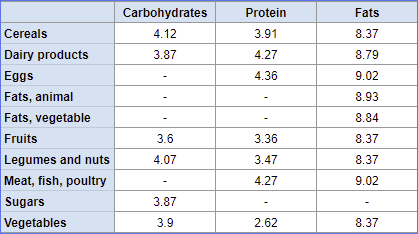How Carb Manager calculates energy (calorie or kilojoule) and macro goals
• 28 May 2021
• Dark
Light
• PDF

# How Carb Manager calculates energy (calorie or kilojoule) and macro goals

• Dark
Light
• PDF

Article Summary

This article describes how Carb Manager’s Macros Calculator determines your energy (calorie or kilojoule) goals and macro goals based on your profile information and the diet choices you make.

When you first start using Carb Manager, you’re prompted to answer questions about your profile (age, sex, height, weight, and activity level) and your goals. When finished, you’re taken to the Macros Calculator, where you refine your energy goal by choosing to lose weight, maintain weight, or build muscle. Lastly, you choose the type of diet to follow, which will determine your macros (carbs, protein, fats) goals.

If you skipped this process previously, or want to revisit your profile and goals, see Create your profile and Set your main diet goals with the Macros Calculator.

Before finalizing your goals, you may want to change your energy setting from calories, the default, to kilojoules.

## Calculating calorie or kilojoule goals

Carb Manager’s Macros Calculator uses the following methods to calculate your energy (calorie or kilojoule) goals which are then used to calculate your macros goals.

Note: One calorie equals 4.184 kilojoules. If you choose to track energy as kilojoules instead of calories, Carb Manager multiplies your calories by 4.184 when calculating energy budgets and goals.

We use the Mifflin - St. Jeor formula for resting metabolic rate (RMR)  (which is sometimes referred to as basal metabolic rate [BMR]) to determine the amount of energy that a person expends per day while at rest.

Formula for men:
(10 multiplied by weight in KG) plus (6.25 multiplied by height in CM) minus (5 multiplied by age) plus 5

Formula for women:
(10 multiplied by weight in KG) plus (6.25 multiplied by height in CM) minus (5 multiplied by age) minus 161

Then, Carb Manager multiples the total energy you burn while at rest by one of the following factors, which are based on your activity level.

• Sedentary: 1.2
• Lightly active: 1.375
• Moderately active: 1.55
• Very active: 1.725
• Extra active: 1.9

The result is the total daily energy expenditure (TDEE) estimate needed to maintain your current weight (maintenance goal).

If you choose to lose weight, your daily energy goal is determined by subtracting the calorie or kilojoule deficit from your maintenance goal.
If you choose to build muscle, your daily energy goal is determined by adding the calorie or kilojoule surplus to your maintenance goal.

We calculate the calorie deficit, or surplus, using the calculation:
3,500 calories multiplied by each pound of body fat you’ve specified in your monthly weight loss, or muscle gain, goal divided by 30.42, the average number of days in a month. Therefore, if x equals pounds of body fat, the equation is (3,500 * x) / 30.42 = calories deficit or surplus.

If you’ve specified kilojoules as your energy unit preference, Carb Manager will automatically convert the values for you.

The calorie or kilojoule deficit or surplus is shown in the macros calculator as a percentage.Tip: To customize your calorie or kilojoule goal, select and move the dial left or right, or selectEdit next to the number of daily calories or kilojoules, enter a number for your goal, and select Submit.

## Example: Calculating calorie or kilojoule goals

A 6 foot tall (183 CM), lightly active, 40-year-old man, weighing 200 lbs (90.72 KG), wants to lose about 4 pounds (1.8 KG) per month.

The formula for men: (10 multiplied by weight in KG) plus (6.25 multiplied by height in CM) minus (5 multiplied by age) plus 5, becomes this RMR:

(10 * 90.72) + (6.25 * 183) - (5 * 40) + 5 = 1,856 RMR

Carb Manager then multiplies the RMR by the factor of 1.375  based on his activity level to determine the total daily energy expenditure (TDEE) estimate needed for a weight maintenance goal:

(1,855.95 * 1.375) = 2,552 TDEE

Finally, to arrive at the daily energy goal, we calculate the calorie deficit (3,500 calories multiplied by four pounds of body fat specified as the monthly weight loss goal divided by 30.42, the average number of days in a month) and subtract it from the TDEE:

(3,500 * 4) / 30.42  = 460 calorie deficit
2,552 - 460 = 2092 calories or 8,753 kilojoules energy budget per day.

## Calculating calories or kilojoules for macronutrients

To calculate your macros (carbs, protein, fats) goals, we use your energy budget (calories or kilojoules) and the type of diet you choose.

Carb Manager uses Atwater’s general energy factors, known as the 4:4:9 method, to calculate the number of calories per gram of each macronutrient:

• Carbohydrates: 4 calories per gram
• Protein: 4 calories per gram
• Fat: 9 calories per gram

## Example: calculating calories or kilojoules for macronutrients

Let’s say the 6 foot tall (183 CM), lightly active, 40-year-old man, weighing 200 lbs (90.72 KG) who wants to lose 4 pounds (1.8 KG) per month, as in the example used above, decides he will follow the Keto diet.

The Keto diet ratios are 5% carbs, 25% protein, and 70%, so taking his calorie budget of 2,093, we break it down using those ratios:

2,092 calories (or 8,753 kj) multiplied by 0.05 equals 105 calories (439 kj) from net carbs
2,092 calories (or 8,753 kj) multiplied by 0.25 equals 523 calories (2,188 kj) from protein
2,092 calories (or 8,753 kj) multiplied by 0.70 equals 1,464 calories (6,125 kj) from fats

Using the 4:4:9 method, we know that net carbs and protein equal 4 calories per gram and fats equals 9 calories per gram, so now we arrive at the macros goals like this:

105 calories (439 kj) from net carbs divided by 4 calories (16.736 kj) equals 26g net carbs
523 calories (2,188 kj) from protein divided by 4 calories (16.736 kj) equals 131g protein
1,464 calories (6,125 kj) from fat divided by 9 calories (37.656 kj) equals 163g fat

Finally, using our fictitious example, the man’s daily goals are:

• 26g net carbs
• 131g protein
• 163g fats
• 2,092 calories (8,753 kj)## Macronutrient share of energy in foods consumed doesn’t always conform to 4:4:9

If you notice that the number of calories or kilojoules per gram of each macronutrient you've consumed and logged in Carb Manager doesn't precisely conform to the 4:4:9 method, that's because food manufacturers in the United States use six different variations in calculating calories from macros: the 4:4:9 method, 4:4:9 minus insoluble fiber, Atwater specific conversion factors for food groups, specific factors approved by the United States Food and Drug Administration (FDA), and other techniques.

For calorie counts, Carb Manager uses the manufacturer's nutrition labels for branded foods and the U.S. Department of Agriculture (USDA) testing for common foods, such as meats and vegetables. The USDA uses Atwater conversion factors, a more precise method of calculating calories from macros than the general Atwater 4:4:9 formula. For more information, see the chart below, the U.S. federal regulations for food labeling, and the University of Minnesota Nutrition Coordinating Center.As a result of these varying methods for deriving calories from macros, the totals you see from the foods you’ve consumed and logged on the daily log may not conform to a 4:4:9 calculation.